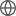Old Web
English
We present a study of strong intervening absorption systems in the near-IR spectra of 31 luminous quasars at $z>5.7$. The quasar spectra were obtained with {\it Gemini} GNIRS that provide continuous wavelength coverage from $\sim$0.9 to $\sim$2.5 $\mu$m. We detect 32 strong Mg II doublet absorbers with rest-frame equivalent width $W_r$ ($\lambda2796$) $>1.0$ Aat $2.2 3$, consistent with previous studies. Compared with strong Mg II absorbers detected in damped Ly$\alpha$ systems at 2 \$